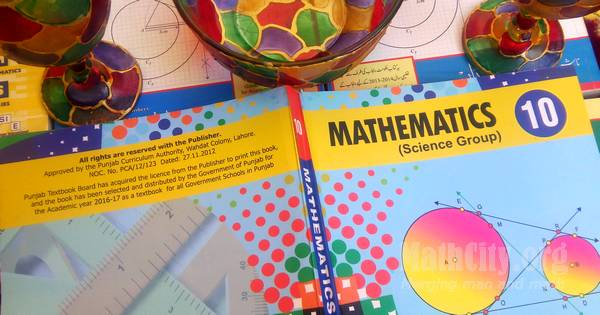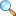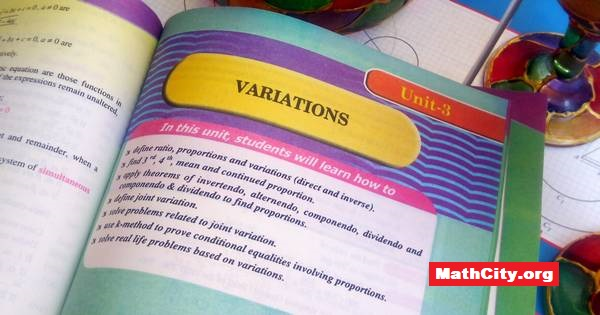# Mathematics 10 (Science Group)The notes/solutions, definitions, MCQs and important question for Mathematics 10 (Science Group), published by Ilmi Kitab Khana, Urdu Bazar, Lahore, Pakistan are available on this page. Whenever we found the notes we will update this page and will upload notes here. If you wish to contribute and send us the notes please contact us via our About Us page.

# Definitions

On this page, definitions from the book “Mathematics 10 (Science Group) (Matric)” are given.

These notes are send by Ms. Amir Shehzad, we are very thankful to him for sending these notes.

In this unit students will learn how to

• solve a quadratic equation in one variable by factorization
• solve a quadratic equation in one variable by completing square.
• derive quadratic formula by using method of completing squaire.

# Unit 02: Theory of Quadratic Equations

These notes are send by Mr. Amir Shehzad, we are very thankful to him for sending these notes.

In this unit students will learn how to

• define discriminant $(b^2-4ac)$ of quadratic expression $ax^2+bx+c$.
• find discriminant of a given quadratic equation.
• discuss the nature of roots of a quadratic equation through discriminant and verify the results by solving the equation.
• determine the nature of the roots of a given quadratic equation and verify the result by solving the equation.
• Exercise 2.8 |View Online | Download PDFThese notes are send by Ms. Maryam Jabeen, we are very thankful to her for sending these notes.

In this unit students will learn how to

• define ratio, proportions and variations (direct and inverse).
• find 3rd, 4th, mean and continued proportion.
• apply theorems of invertendo, alternendo, componendo, dividendo and compnendo and dividendo to find proportions
• define joint variation.
• solve problems related to joint variation.
• use k-method to prove conditional equalities involving proportions.
• solve real life problems based on variations.

The following notes are sent by Mr. Amir Shehzad. We are very thankful to him.

• Unit 03 |View Online | Download PDFThese notes are send by Ms. Maryam Jabeen, we are very thankful to her for sending these notes.

In this unit, students will learn how to

• define proper, improper, rational fraction.
• resolve an algebraic fraction into partial fractions, when its denominator consist of
• non-repeated linear ffactor,
• repeated linear factor,

The following notes are sent by Mr. Amir Shehzad. We are very thankful to him.

• Unit 04 |View Online | Download PDFThese notes are send by Mr. Amir Shehzad, we are very thankful to him for sending these notes.

In this unit students will learn how to

• sets
• recall the sets denoted by $\mathbb{N}$, $\mathbb{W}$, $\mathbb{Z}$, $E$, $O$, $P$ and $\mathbb{Q}$.
• recognize operation on sets ($\cup$, $\cap$, $\setminus$,…)
• perform operations in set union, intersection, difference, complement.
• give formal proofs of the following fundamental properties of union and intersection of two or three sets
• commutative property of union.
• commutative property of intersection.
• associative property of union.
• associative property of intersection.
• Exercise 5.2 |View Online | Download PDFIn this unit students will learn:

• how to prove the following theorems alongwith corollaries and apply them to solve appropriate problems
• One and only one circle can pass through three non collinear points.
• A straight line drawn from the centre of a circle to bisect a chord (which is not a diameter) is perpendicular to the chord.
• Perpendicular from the centre of a circle on a chord bisects it.
• Two chords of a circle which are equidistant from the centre are congruent.

The following theorems was send by Bahadar Ali Khan. We are very thankful to him for sending these notes.

• matric/10th_science